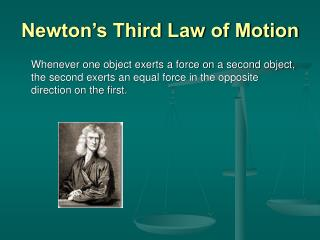Download PresentationNewton’s Third Law of MotionNewton’s Third Law of Motion - PowerPoint PPT Presentation

Download PresentationNewton’s Third Law of Motion
An Image/Link below is provided (as is) to download presentation

Download Policy: Content on the Website is provided to you AS IS for your information and personal use and may not be sold / licensed / shared on other websites without getting consent from its author. While downloading, if for some reason you are not able to download a presentation, the publisher may have deleted the file from their server.

- - - - - - - - - - - - - - - - - - - - - - - - - - - E N D - - - - - - - - - - - - - - - - - - - - - - - - - - -
Presentation Transcript

1. Newton’s Third Law of Motion Whenever one object exerts a force on a second object, the second exerts an equal force in the opposite direction on the first.

2. First Example of 3rd Law A horse harnessed to a cart exerts an equal and opposite force to the cart as it exerts a force against the ground.

3. Second Example of 3rd Law Space shuttle’s rocket boosters propel the orbiter into space by exerting an equal and opposite force to exhaust gasses.

4. Concept Question 1 Why are we able to walk?

5. Concept Question Answer We walk forward because when one foot pushes backward against the ground, the ground pushes forward on that foot. Force exerted on the person’s foot by the ground. Fpg Force exerted on the ground by person’s foot. Fgp Fgp=-Fpg

6. Concept Question 2 What makes a car go forward?

7. Concept Question answer By Newton’s third law, the ground pushes on the tires in the opposite direction, accelerating the car forward.

8. Concept Question Which is stronger, the Earth’s pull on an orbiting space shuttle or the space shuttle’s pull on the earth?

9. Concept Question Answer According to Newton’s Third Law, the two forces are equal and opposite. Because of the huge difference in masses, however the space shuttle accelerates much more towards the Earth than the Earth accelerates toward the space shuttle. a = F/m

10. Problem 1 What force is needed to accelerate the 60 kg cart at 2m/s^2?

11. How to solve Problem 1 What force is needed to accelerate the 60kg cart at 2 m/s^2? Force = mass times acceleration F = m * a F = 60kg * 2m/s^2 F = 120kgm/s^2 Kgm/s^2 = Newton Newton = N F =120 N

12. Problem 2 A force of 200 N accelerates a bike and rider at 2 m/s^2. What is the mass of the bike and rider?

13. How to solve Problem 2 • A force of 200 N accelerate a bike and rider at 2m/s^2. What is the mass of the bike and rider? F = ma therefore:m =F/a m = 200N/2m/s^2 N= kgm/s^2 so when divide your answer will be kg left. m = 100kg